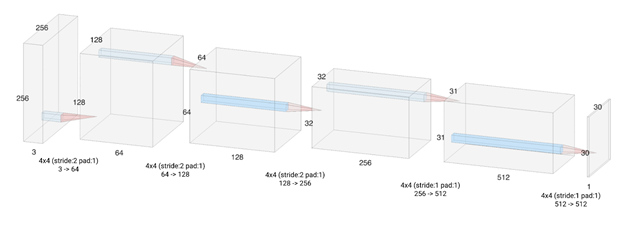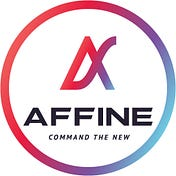# PIX2PIX — Machine Learning Tool for Artists

As an artist, I always wondered if I could bring my art to life. Although, it makes no sense, what if I told you that this was possible with Machine Learning? Imagine a machine learning algorithm that can convert all your sketches with a simple line of your drawing as a reference point to convert this into an oil painting based on its understanding of real-world shapes and patterns from human drawings, and photos. As an accomplished artist, your results can be quite interesting.

Take a look at the example below:

Pix2Pix is a Generative Adversarial Network, or GAN model designed for general purpose image-to- image translation. Image to Image translation is a problem where you have to translate a given image domain to a target domain. For example, let’s say the input domain images are of cats, and the target domain images are of dogs. In this case, the Image-to-Image translation algorithm learns mapping from inputs to the target domain in such a way that if you input the image of a dog, it can change it to an image of a cat.

# Pix2pix can also be used to:

• Convert satellite imagery into a Google Maps-style street view
• Translate images from daytime to nighttime
• Sketch products to product photographs. For e.g., for shoe commercials
• Convert high intensity images into low intensity and vice-versa

Pix2Pix algorithm is one of the first successful general Image-to-Image translation algorithms that use “Gan Loss” to generate realistic image outputs. It is shorthand for an implementation of a generic image- to-image translation using conditional adversarial networks.

Compared to other GAN models for conditional image generation, pix2pix is relatively simple and capable of generating large, high-quality images across a variety of image translation tasks.

The comparison below should give you an idea of its potential:

The GAN architecture is comprised of a Generator Model for outputs of new plausible synthetic images, and a Discriminator Model that classifies images as Real (from the dataset) or Fake (generated). The discriminator model is updated directly, whereas the generator model is updated via the discriminator model, and the two models are trained simultaneously in an adversarial process where the generator seeks to better fool the discriminator where the discriminator seeks to better identify the counterfeit images.

The Pix2Pix model is a type of conditional GAN, or cGAN, where the generation of the output image is conditional based on the input, and in this case, it is a source image. The discriminator is provided with a source image, and the target image; the model must determine whether the target is a plausible transformation of the source image.

The Generator’s Network
Generator network uses a U-Net-based architecture. U-Net’s architecture is similar to an Auto- Encoder network as it uses Encoder and the Decoder for processing .

• U-Net’s network has skip connections between Encoder layers and Decoder layers.
• As shown in the picture, the output of the first layer of Encoder is directly passed to the last layer of the Decoder, and output of the second layer of Encoder is pass to the second last layer of
the Decoder and so on.
• if there are total N layers in U-Net’s (including middle layer), then there will be a skip connection from the kth layer in the Encoder network to the (N-k+1)th layer in the Decoder network. where 1
≤ k ≤ N/2.

‘x’ and ‘y’ represent input and output channels, respectively.

# The Generator’s Architecture

## The Generator network is made up of these two networks:

• The Encoder network is a downsampler
• The Decoder network is an upsampler

## The Generator’s Encoder Architecture

• The Encoder network of the Generator network has seven convolutional blocks
• Each convolutional block has a convolutional layer, followed by a Leaky ReLU activation function
• Each convolutional block also has a batch normalization layer except for the first layer

## The Generator’s Decoder Architecture

• The Decoder network of the Generator network has seven upsampling convolutional blocks
• Each upsampling block has an upsampling layer, followed by a convolutional layer, a batch of normalization layer and a ReLU activation function.

## There are six skip-connections in a Generator network. The concatenation happens along the channel axis:

• The output from the 1st Encoder block is concatenated to the 6th Decoder block.
• The output from the 2nd Encoder block is concatenated to the 5th Decoder block.
• The output from the 3rd Encoder block is concatenated to the 4th Decoder block.
• The output from the 4th Encoder block is concatenated to the 3rd Decoder block.
• The output from the 5th Encoder block is concatenated to the 2nd Decoder block.
• The output from the 6th Encoder block is concatenated to the 1st Decoder block.

## Discriminator’s Architecture

Discriminator network uses of PatchGAN architecture. The PatchGAN network contains five convolutional blocks.Figure 1 PatchGAN Architecture with 5 convolutional Network

## Pix2Pix Network’s Training

Pix2Pix is a conditional GANs. The loss function for the conditional GANs can be written as below:

# Following are the steps that involve training the model for the Pix2Pix algorithm:

## 4. Build the Generator

• The architecture of generator is a modified U-Net.
• Each block in the encoder is (Conv -> Batchnorm -> Leaky ReLU)
• Each block in the decoder is (Transposed Conv -> Batchnorm -> Dropout (applied to the first three blocks) -> ReLU)
• There are skip connections between the encoder and decoder (as in U-Net).

## 5. Generator loss

• It is a sigmoid cross entropy loss of the generated images and an array of ones
• It includes L1 loss which is MAE (mean absolute error) between the generated image and the target image
• This allows the generated image to become structurally similar to the target image
• The formula to calculate the total generator loss = gan_loss + LAMBDA * l1_loss, where LAMBDA
= 100 l

The training procedure for the generator is shown below:

## 6. Build the Discriminator

• The Discriminator is a PatchGAN.
• Each block in the discriminator is (Conv -> BatchNorm -> Leaky ReLU)
• The shape of the output after the last layer is (batch_size, 30, 30, 1)
• Each 30x30 patch of the output classifies a 70x70 portion of the input image (such an architecture is called a PatchGAN).
• Discriminator receives 2 inputs:
o Input image and the target image, which it should classify as real.
o Input image and the generated image (output of the generator), which it should classify as fake.
o We concatenate these 2 inputs together in the code (tf.concat([inp, tar], axis=-1))

## 7. Discriminator loss

• The discriminator loss function takes 2 inputs: real images and generated images
• real_loss is a sigmoid cross entropy loss of the real images and an array of ones (since these are the real images)
• generated_loss is a sigmoid cross entropy loss of the generated images and an array of zeros
(since these are the fake images)
• Then the total_loss is the sum of real_loss and the generated_loss

The training procedure for the discriminator is shown below:

## 9. Generate Images

Write a function to plot some images during training.

• We pass images from the test dataset to the generator
• The generator will then translate the input image into the output
• Last step is to plot the prediction

## 10. Training

• For each example input, generate an output
• The discriminator receives the input_image and the generated image as the first input. The second input is the input_image and the target_image
• Next, we calculate the generator and the discriminator loss
• Then, we calculate the gradients of loss with respect to both the generator and the discriminator variables (inputs) and apply those to the optimizer
• Then log the losses to TensorBoard

## The Training Loop:

• Iterates over the number of epochs
• On each epoch, it clears the display, and runs generate_images to show its progress
• On each epoch it iterates over the training dataset, printing a ‘.’ for each example
• It saves a checkpoint every 20 epochs

The beauty about a trained pix2pix network is that it will generate an output from any arbitrary input. Following are the inputs and their corresponding outputs generated after applying Pix2Pix.

Input

Output

Following are some other Image transformations by Pix2Pix:

# Conclusion

Pix2Pix is a whole new strategy for Image-to-Image translation using a combination of the Generator and Discriminator. It gives us chance to turn our art into life. It also proves to be useful in various spheres like exploring satellite images and in various Augment Reality techniques. This technique could open a new opportunity for Virtual Reality and give it a whole new approach.

## Reference

Network_CVPR_2019_paper.pdf

Image_Translation_With_CVPR_2017_paper.pdf

This Blog is written by Anamika Jha, Business Analyst, Affine.

--

--## Affine

Affine is a provider of analytics solutions, working with global organizations solving their strategic and day to day business problems www.affineanalytics.com